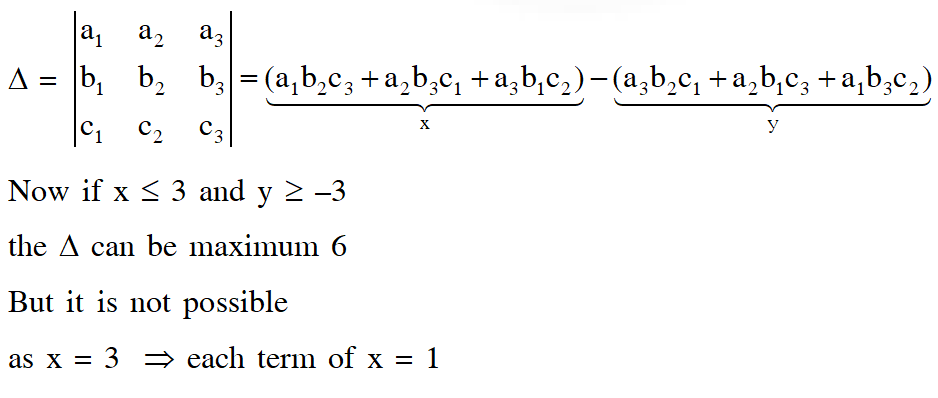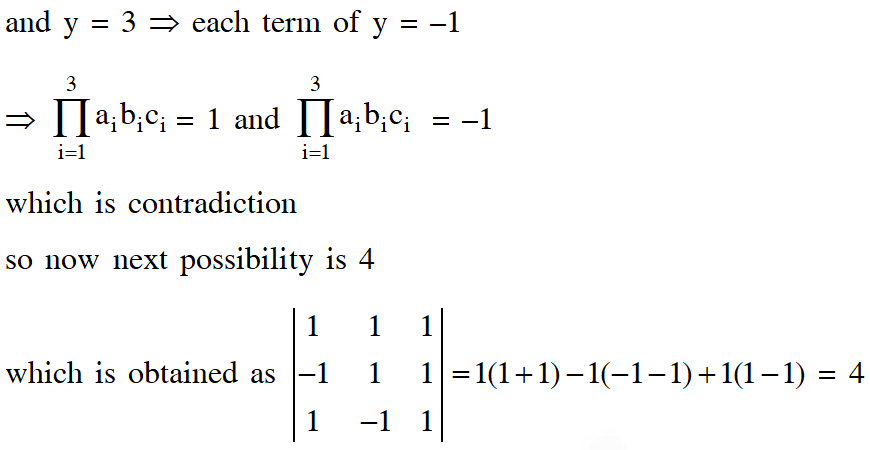# Determinant - JEE Advanced Previous Year Questions with Solutions

JEE Advanced Previous Year Questions of Math with Solutions are available at eSaral. Practicing JEE Advanced Previous Year Papers Questions of mathematics will help the JEE aspirants in realizing the question pattern as well as help in analyzing weak & strong areas. eSaral helps the students in clearing and understanding each topic in a better way. eSaral also provides complete chapter-wise notes of Class 11th and 12th both for all subjects. Besides this, eSaral also offers NCERT Solutions, Previous year questions for JEE Main and Advance, Practice questions, Test Series for JEE Main, JEE Advanced and NEET, Important questions of Physics, Chemistry, Math, and Biology and many more. Download eSaral app for free study material and video tutorials.
Q. The number of all possible values of $\theta,$ where $0<\theta<\pi,$ for which the system of equations $(\mathrm{y}+\mathrm{z}) \cos 3 \theta=(\mathrm{xyz}) \sin 3 \theta \mathrm{x} \sin 3 \theta=\frac{2 \cos 3 \theta}{\mathrm{y}}+\frac{2 \sin 3 \theta}{\mathrm{z}}(\mathrm{xyz}) \sin 3 \theta=(\mathrm{y}+2 \mathrm{z}) \cos 3 \theta+\mathrm{ysin} 3 \theta$ have a solution $\left(\mathrm{x}_{0}, \mathrm{y}_{0}, \mathrm{z}_{0}\right)$ with $\mathrm{y}_{0} \mathrm{z}_{0} \neq 0,$ is [JEE 2010,3]
Ans. 3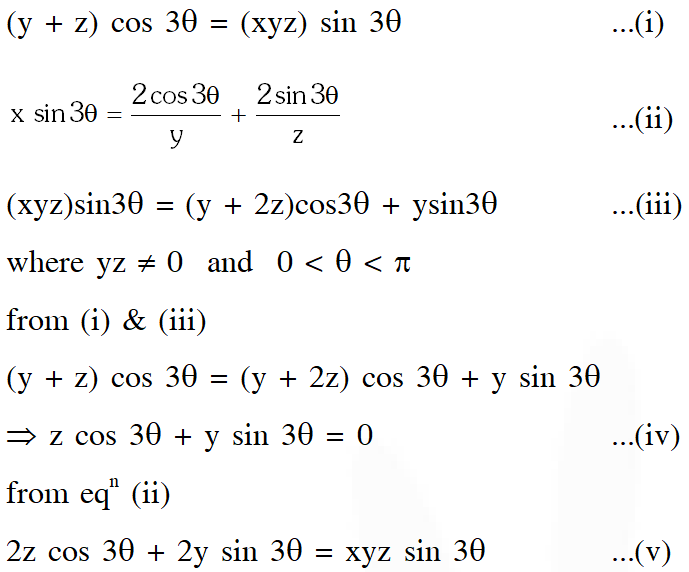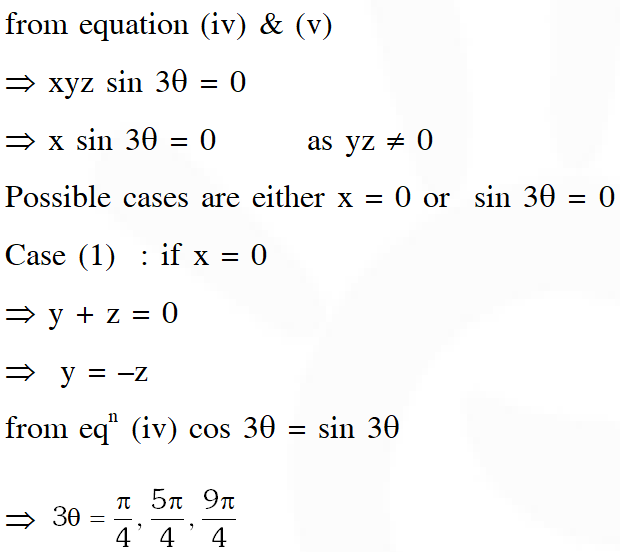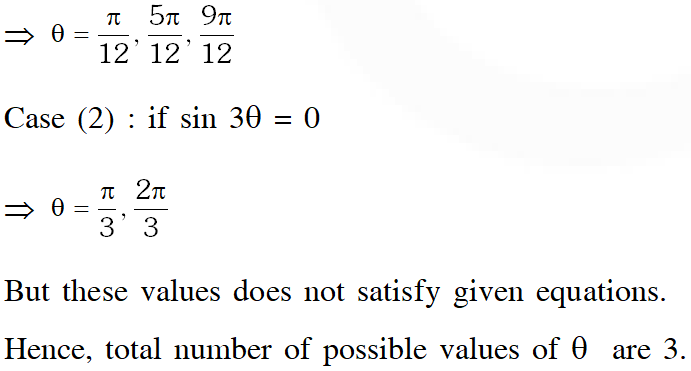Q. Which of the following values of  satisfy the equation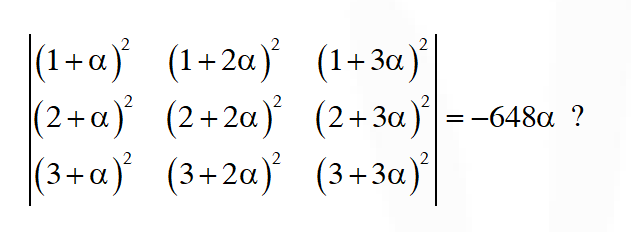(A) –4            (B) 9              (C) –9                (D) 4 [JEE(Advanced)-2015, 4M, –2M]
Ans. (B,C)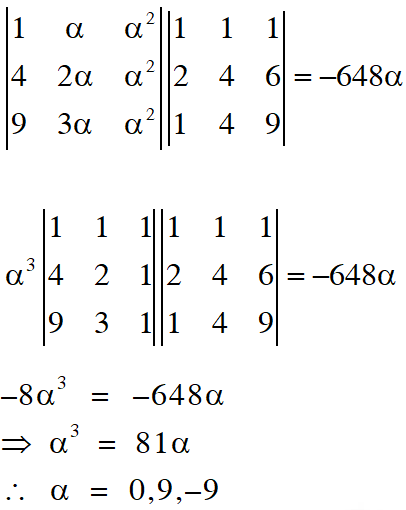Q. Let a, $\lambda, \mathrm{m} \in \square .$ Consider the system of linear equations $a x+2 y=\lambda$ $3 x-2 y=\mu$ Which of the following statement(s) is(are) correct? (A) If $a=-3,$ then the system has infinitely many solutions for all values of $\lambda$ and $\mu$ (B) If $a \neq-3,$ then the system has a unique solution for all values of $\lambda$ and $\mu$ (C) If $\lambda+\mu=0,$ then the system has infinitely many solutions for $a=-3$ (D) If $\lambda+\mu \neq 0,$ then the system has no solution for $a=-3$ [JEE(Advanced)-2016]
Ans. (B,C,D)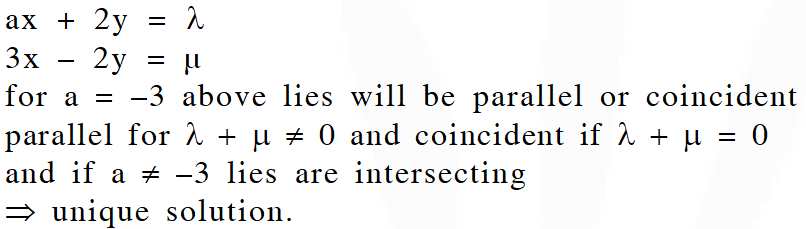Q. The total number of distinct $\mathrm{x} \in \mathrm{R}$ for which $\left|\begin{array}{ccc}{\mathrm{x}} & {\mathrm{x}^{2}} & {1+\mathrm{x}^{3}} \\ {2 \mathrm{x}} & {4 \mathrm{x}^{2}} & {1+8 \mathrm{x}^{3}} \\ {3 \mathrm{x}} & {9 \mathrm{x}^{2}} & {1+27 \mathrm{x}^{3}}\end{array}\right|=10$ is [JEE(Advanced)-2016]
Ans. 2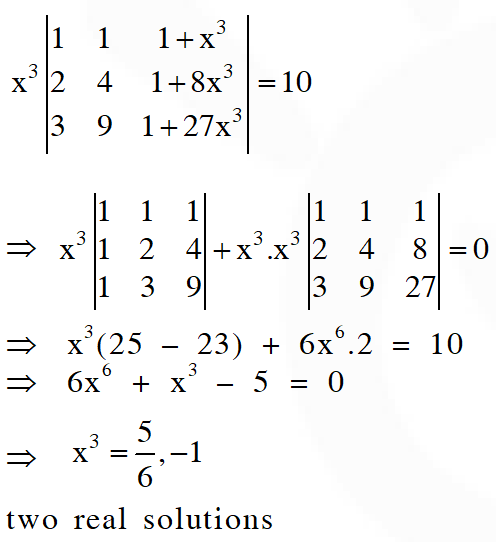Q. Let $\mathrm{P}$ be a matrix of order $3 \times 3$ such that all the entries in $\mathrm{P}$ are from the set $\{-1,0,1\} .$ Then, the maximum possible value of the determinant of $\mathrm{P}$ is [JEE(Advanced)-2018]
Ans. 4